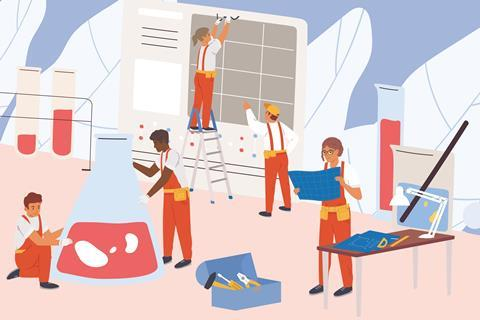Fix students’ learning with purpose and a ‘broken’ approachCan we fix it? Yes, we can. Use the broken practical approach to deepen students’ learning from experiments

Practical work is a key feature of science teaching. Since the introduction of core, or required, practicals into GCSE courses I believe the quantity of practical work has increased. However, is this increase in quantity having the desired effect in terms of student learning? Is the quality of these lessons as good as it could be?

When we plan lessons, we should focus on what we want our students to learn rather than on whether they can complete a particular task. Practical work has to be the same – the aim of a lesson must be to learn something, not simply to carry out a procedure. Of course, the clearer the aim and the better it is communicated to students, the more likely the practical activity is to be successful.

## Identify the purpose

So what is the purpose of practical work? It varies from lesson to lesson, and between age groups. If we concentrate on those practicals required by exam boards, the specification will help identify the purpose; for GCSEs that’s the ‘apparatus and techniques’ section. Looking at the exam spec tells you what experience the practical work has been designed to give students and shows why the exam boards chose those particular practicals. This knowledge will help you plan and focus student attention on that area when carrying out the practical. Assessment within and after the practical activity should be aimed at these specification points rather than just checking a student can recite the procedure and results. Ask questions that assess the students’ understanding of the apparatus and techniques being developed. You can use the ‘broken practical approach’ described below for this.

When planning a practical lesson, taking a longer view ensures students get the most from the work. Consider the lessons preceding the practical lesson. How do they build the experience and develop a level of understanding that will prepare students for the practical? This doesn’t just have to be in the lessons immediately preceding.

A ‘broken practical’ question matrix to better explore students’ understanding of their practical work as MS Word or pdf.

A ‘broken practical’ question matrix to better explore students’ understanding of their practical work from the Education in Chemistry website: rsc.li/3eUQRqr

In my department we have looked back to key stage 3, considering how we can incorporate the apparatus and techniques from the GCSE specification. This does not mean we do the required practical earlier. Instead it means introducing the equipment and procedures in a different context with the appropriate support and guidance. This supports students’ understanding and performance in the final exams; for GCSE, that’s AO1–3.

In effect, we are working on demonstrating knowledge and understanding of scientific ideas, techniques and procedures (AO1) with 11–14-year-old students so that they can recall and identify things accurately. Then we use the practicals themselves with 14–16 students to develop the understanding required to apply that knowledge (AO2) so they can answer exam questions testing their abilities to analyse information and ideas, interpret and evaluate, make judgements, draw conclusions and improve experimental procedures (AO3). Having that solid experience from earlier on helps them to do this well.

## Take the broken approach

When we are developing and assessing our students’ ability to understand the practical work they are doing rather than just their ability to follow recipe-style instructions, I find a ‘broken practical’ approach works well. This moves from recall questions about what students did to more challenging, in-depth questions exploring what students are learning. You can use a questioning matrix to develop your questions (download an example matrix below). The idea is that by changing the first few words of a question, you significantly change the level and challenge of that question. To find out how much students really understand, use plenty of questions from the bottom right of the matrix.

For example, with students who’ve completed the chromatography of ink, rather than asking them to recall where they drew the pencil line, you could ask: Why should the line be drawn with a pencil? Or what might they use if they didn’t have a pencil? This style of question requires them to analyse and evaluate the practical, developing deeper understanding.

By focusing the planning of practical lessons on the skills and understanding we want students to develop, and by assessing these during and after the lesson, we can be more confident that practical work has a positive impact on students’ learning. By breaking away from the recipe-style practical, we can get more value from the practicals taking place in our classrooms.